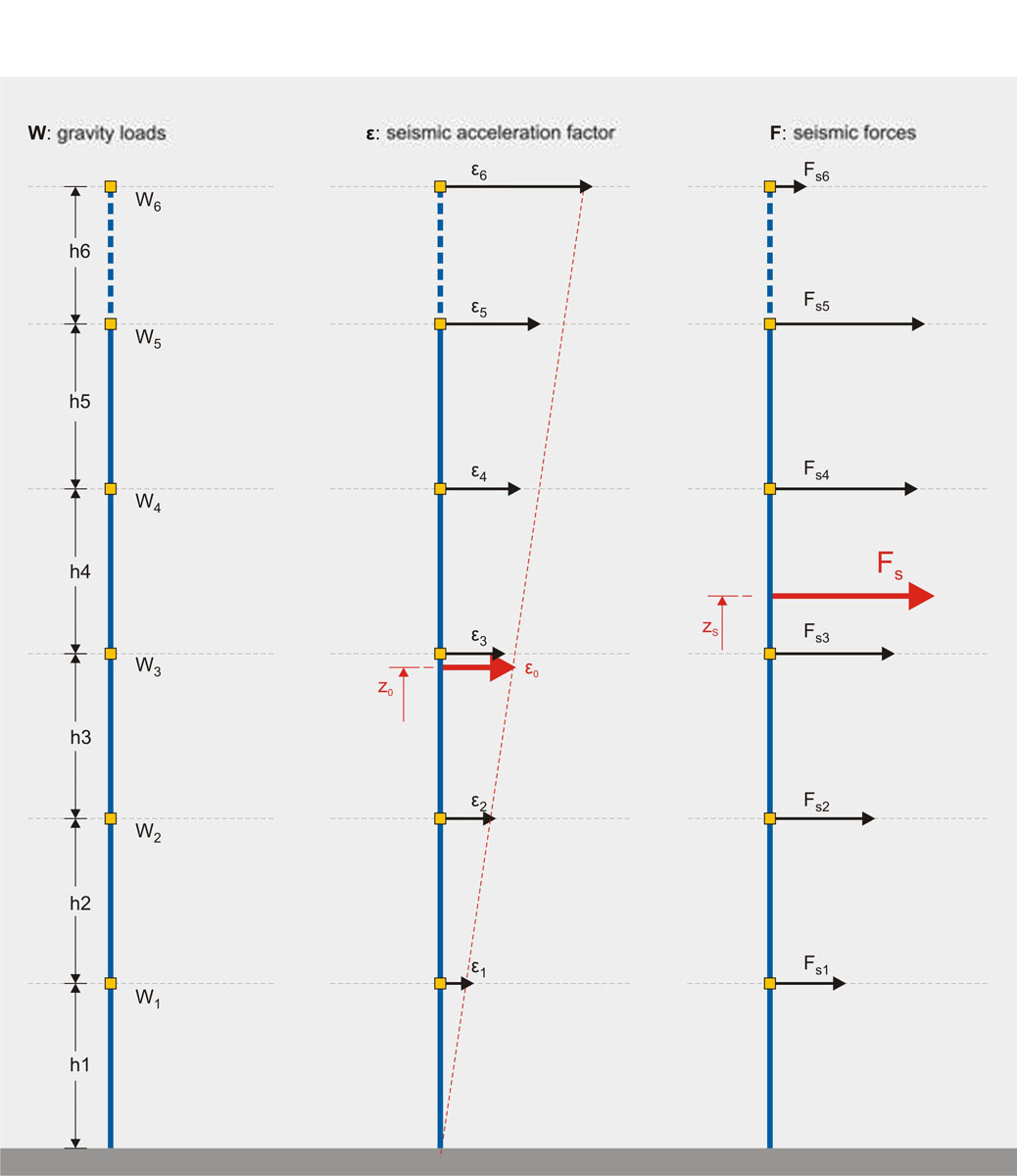« Gravity Loads Wind loading » Seismic loads The effect that a seismic action will have in a structure is determined by regarding an earthquake with horizontal design ground seismic acceleration equal to A=α*g. Greece is divided into three Seismic Zones Z1, Z2, Z3 and for each of these Seismic Zones, for structures on ground A quality, (adequate ground) the factor α takes the following values 0.16 for Z1, 0.24 for Z2 and 0.36 for Z3. In the duration of an earthquake, a horizontal seismic force H is applied in every mass M that has gravity load W. The force H is equal to a percentage ε of the force W. ε value usually varies between 0.00 and 0.50 while in a very intense seismic action it may rise above 1.00. The value of ε depends mainly upon the value of factor α, used for the calculation of the maximum horizontal ground seismic acceleration and upon other factors like soil classification, structural system’s geometry, mass center. In general terms, the distribution of seismic accelerations has the form of a triangle.The distribution of seismic acceleration has a form similar to a triangle The distribution of seismic acceleration has a form similar to a triangle The structure shown above belongs to the Seismic Zone Z1 (seismic ground acceleration equal to 0.16), the mean value of the design seismic acceleration is around 0.12g (ε0=0.12) and the resultant seismic force Fs is around 1400 kN. The theoretical height that this force is applied to, is around the 2/3 of the structure’s total height. « Gravity Loads Wind loading »